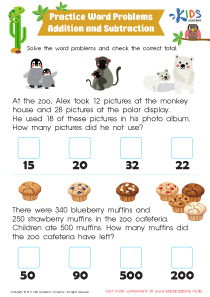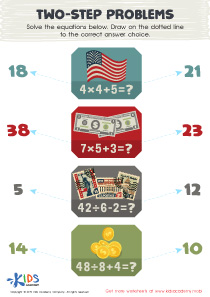# Math Lesson - 2 Step Word Problems Writing Equations to Match, Grade 3

• ### Activity 1 / Addition and Subtraction: Word Problems WorksheetWord problems are tough, but offer yet another way to solve math problems for enhanced math understanding.
This addition and subtraction word problem worksheet for 3rd grade takes your child to the zoo to answer two intriguing word problems that will challenge him to think hard to find the total!

• ### Activity 3 / Two-Step Problems WorksheetAs your child gets introduced to new parts of math, the strain might be a lot for them. Use this worksheet to help them practice their problem solving skills. Some of the earliest things your kids will learn are how to do subtractions, additions, multiplication, and division of numbers. In this tracing sheet, there are four simple equations. Help your kids solve the equations and then draw on the dotted line to the correct answer choice.

• ### Activity 4 / Sightseeing 2-Step Word Problems WorksheetWhen your kids are presented with word problems, they might be a little confused as to how to go about solving the equations. However, with the practice this worksheet affords them, that confusion will soon be a thing of the past. In this PDF, there are two simple word problems. Read them aloud to your kids, and assist them in creating the equation. Then, help them solve the word problems and then check the box under the correct answer.

?